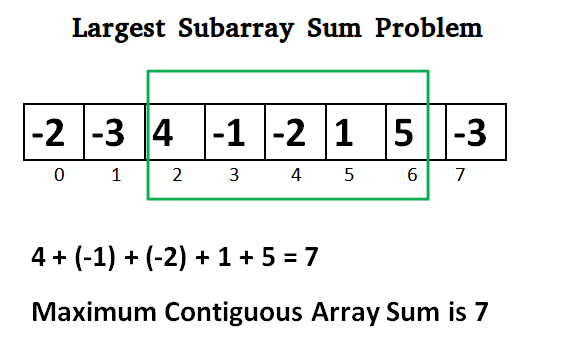# Java Program for Largest Sum Contiguous Subarray

Write an efficient program to find the sum of contiguous subarray within a one-dimensional array of numbers which has the largest sum.## Java

 `import` `java.io.*; ` `// Java program to print largest contiguous array sum ` `import` `java.util.*; ` ` `  `class` `Kadane { ` `    ``public` `static` `void` `main(String[] args) ` `    ``{ ` `        ``int``[] a = { -``2``, -``3``, ``4``, -``1``, -``2``, ``1``, ``5``, -``3` `}; ` `        ``System.out.println(``"Maximum contiguous sum is "` `+ maxSubArraySum(a)); ` `    ``} ` ` `  `    ``static` `int` `maxSubArraySum(``int` `a[]) ` `    ``{ ` `        ``int` `size = a.length; ` `        ``int` `max_so_far = Integer.MIN_VALUE, max_ending_here = ``0``; ` ` `  `        ``for` `(``int` `i = ``0``; i < size; i++) { ` `            ``max_ending_here = max_ending_here + a[i]; ` `            ``if` `(max_so_far < max_ending_here) ` `                ``max_so_far = max_ending_here; ` `            ``if` `(max_ending_here < ``0``) ` `                ``max_ending_here = ``0``; ` `        ``} ` `        ``return` `max_so_far; ` `    ``} ` `} `

Output:

```Maximum contiguous sum is 7
```

Above program can be optimized further, if we compare max_so_far with max_ending_here only if max_ending_here is greater than 0.

## Java

 `static` `int` `maxSubArraySum(``int` `a[], ``int` `size) ` `{ ` ` `  `    ``int` `max_so_far = ``0``, max_ending_here = ``0``; ` ` `  `    ``for` `(``int` `i = ``0``; i < size; i++) { ` `        ``max_ending_here = max_ending_here + a[i]; ` `        ``if` `(max_ending_here < ``0``) ` `            ``max_ending_here = ``0``; ` ` `  `        ``/* Do not compare for all ` `           ``elements. Compare only  ` `           ``when max_ending_here > 0 */` `        ``else` `if` `(max_so_far < max_ending_here) ` `            ``max_so_far = max_ending_here; ` `    ``} ` `    ``return` `max_so_far; ` `} ` ` `  `// This code is contributed by ANKITRAI1 `

Please refer complete article on Largest Sum Contiguous Subarray for more details!

My Personal Notes arrow_drop_up
Article Tags :

Be the First to upvote.

Please write to us at contribute@geeksforgeeks.org to report any issue with the above content.